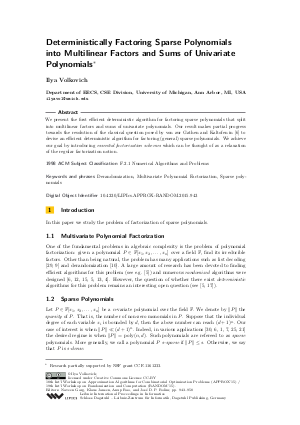Document# Deterministically Factoring Sparse Polynomials into Multilinear Factors and Sums of Univariate Polynomials

### Author Ilya Volkovich## File

LIPIcs.APPROX-RANDOM.2015.943.pdf
• Filesize: 0.52 MB
• 16 pages

## Cite As

Ilya Volkovich. Deterministically Factoring Sparse Polynomials into Multilinear Factors and Sums of Univariate Polynomials. In Approximation, Randomization, and Combinatorial Optimization. Algorithms and Techniques (APPROX/RANDOM 2015). Leibniz International Proceedings in Informatics (LIPIcs), Volume 40, pp. 943-958, Schloss Dagstuhl - Leibniz-Zentrum für Informatik (2015)
https://doi.org/10.4230/LIPIcs.APPROX-RANDOM.2015.943

## Abstract

We present the first efficient deterministic algorithm for factoring sparse polynomials that split into multilinear factors and sums of univariate polynomials. Our result makes partial progress towards the resolution of the classical question posed by von zur Gathen and Kaltofen in [von zur Gathen/Kaltofen, J. Comp. Sys. Sci., 1985] to devise an efficient deterministic algorithm for factoring (general) sparse polynomials. We achieve our goal by introducing essential factorization schemes which can be thought of as a relaxation of the regular factorization notion.
##### Keywords
• Derandomization
• Multivariate Polynomial Factorization
• Sparse polynomials

## Metrics

• Access Statistics
• Total Accesses (updated on a weekly basis)
0